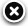# NavList:

## A Community Devoted to the Preservation and Practice of Celestial Navigation and Other Methods of Traditional Wayfinding

Message:αβγ
Message:abc
 Add Images & Files Name or NavList Code: Email:
Re: Instumental error?
From: Gary LaPook
Date: 2005 Apr 20, 14:24 -0700
When calculating standard deviation for some types of data you divide by "n" and for others "n-1" e.g.6 in this case. You use the "n" when calculating for a whole population. You use "n-1" when computing from a sample of the population because in this case using just "n" results in a biased standard deviation.Standard DeviationThe standard deviationof a probability distribution is defined as the square root of the variance,(1)(2)

whereis the mean,is the second raw moment, anddenotes an expectation value. The varianceis therefore equal to the second central moment (i.e., moment about the mean),(3)

The square root of the sample variance of a set of N values is the sample standard deviation(4)

The sample standard deviation distribution is a slightly complicated, though well-studied and well-understood, function.

However, consistent with widespread inconsistent and ambiguous terminology, the square root of the bias-corrected variance is sometimes also known as the standard deviation,(5)

Given only a sample of values x1,...,xn from some larger population, many authors define the sample standard deviation by$s = \sqrt{\frac{1}{n-1} \sum_{i=1}^n (x_i - \overline{x})^2} .$

A slightly faster way to compute the same number is given by the formula$s = \sqrt{\frac{n\sum_{i=1}^n{{x_i}^2} - \left(\sum_{i=1}^n{x_i}\right)^2}{n \, (n-1)}\ } .$

The reason for this definition is that s2 is an unbiased estimator for the variance σ2 of the underlying population. (The derivation of this equation assumes only that the samples are uncorrelated and makes no assumption as to their distribution.) Note however that s itself is not an unbiased estimator for the standard deviation σ; it tends to underestimate the population standard deviation. Although an unbiased estimator for "s" is known, the formula is overly complicated and amounts to a minor correction. Moreover, unbiasedness, in this sense of the word, is not always desirable; see bias (statistics). Some have even argued that the difference between n and n − 1 in the denominator is overly complex and trivial and thus exclude it. Without that term, what is left is the simpler expression

George Huxtable wrote:
Alex wrote-


I measure some distance several times, say 7 times in 10 minutes.
A star-to star distance can be considered constant for this period.
The average is the arithmetic average.
(x_1+x_2+x_3+x_4+x_5+x_6+x_7)/7 in this case.
Here x_1...x_7 are my sextant readings.
The standard deviation.
I subtract the average from each x_j, square the difference
add the results, divide by 6 and extract the
square root. (Actually the calculator does it all for me).


====================

One thing puzzles me. Why, in finding the mean of 7 squared-deviations from
the average, does Alex (or his calculator) sum them and then divide by 6
rather than by 7?

George.

================================================================
contact George Huxtable by email at george@huxtable.u-net.com, by phone at
01865 820222 (from outside UK, +44 1865 820222), or by mail at 1 Sandy
Lane, Southmoor, Abingdon, Oxon OX13 5HX, UK.
================================================================Browse Files

Drop Files### NavList### What is NavList?### Get a NavList ID Code

 Name: (please, no nicknames or handles) Email:
 Do you want to receive all group messages by email? Yes No
A NavList ID Code guarantees your identity in NavList posts and allows faster posting of messages.### Retrieve a NavList ID Code

Enter the email address associated with your NavList messages. Your NavList code will be emailed to you immediately.
 Email:### Email Settings

 NavList ID Code:### Custom Index

 Subject: Author: Start date: (yyyymm dd) End date: (yyyymm dd)